## Thursday, April 4, 2013

### WP8 Tip: How to Animate a Clipping Mask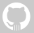Following the post I published yesterday about how to create clipping masks, I want to share techniques to animate them. Animating a clipping mask is a nice way to achieve transitions between elements on your user interface. Lets get started.

First, to be able to animate the clipping mask, you need to have a Transform element attached to it, as you would do with any other object you want to animate. In this example, I’ll be using a TranslateTransform, since we will be moving objects in the two-dimensional x-y coordinate system.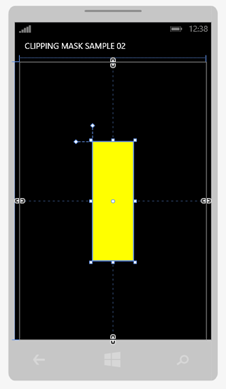So lets start with a simple rectangle with a clipping mask, defined in XAML code as follows:

`  1: <Rectangle HorizontalAlignment="Center"  2:            VerticalAlignment="Center"  3:            Fill="Yellow"  4:            Height="300"  5:            Width="100">  6:     <Rectangle.Clip>  7:         <RectangleGeometry Rect="0, 0, 100, 300"/>  8:     </Rectangle.Clip>  9: </Rectangle>`

Then, lets add the transform element to the RectangleGeometry of the rectangle’s clip, so we can actually move it:

`  1: <Rectangle HorizontalAlignment="Center"  2:            VerticalAlignment="Center"  3:            Fill="Yellow"  4:            Height="300"  5:            Width="100">  6:     <Rectangle.Clip>  7:         <RectangleGeometry Rect="0, 0, 100, 300">  8:             <RectangleGeometry.Transform>  9:                 <TranslateTransform /> 10:             </RectangleGeometry.Transform> 11:         </RectangleGeometry> 12:     </Rectangle.Clip> 13: </Rectangle>`

Now, we can do the animations. But before, lets do a couple of things so we can actually see the effect we want to achieve.

Lets add an underlying rectangle with a different color. That way, we will be able to move the clipping mask to make the yellow rectangle to appear or disappear. Lets add a red rectangle object before our clipped yellow one with the same dimensions. In this case, I’m doing it within a grid container so I can position them one on top of the other.

`  1: <Grid x:Name="ContentPanel"   2:       Margin="12,0,12,0">  3:   4:     <Rectangle HorizontalAlignment="Center"  5:                 VerticalAlignment="Center"  6:                 Fill="Red"  7:                 Height="300"  8:                 Width="100"/>  9:  10:     <Rectangle HorizontalAlignment="Center" 11:                 VerticalAlignment="Center" 12:                 Fill="Yellow" 13:                 Height="300" 14:                 Width="100"> 15:         <Rectangle.Clip> 16:             <RectangleGeometry Rect="0, 0, 100, 300"> 17:                 <RectangleGeometry.Transform> 18:                     <TranslateTransform /> 19:                 </RectangleGeometry.Transform> 20:             </RectangleGeometry> 21:         </Rectangle.Clip> 22:     </Rectangle> 23:  24: </Grid>`
Now lets do a quick test. In the TranslateTransform element, modify the Y property, like this:
`  1: <RectangleGeometry.Transform>  2:     <TranslateTransform Y="100" />  3: </RectangleGeometry.Transform>`

What you are doing is moving the clipping mask of the yellow rectangle down. Since our yellow rectangle is on top of the red one, you will start to see a part of the underlying red rectangle as you move the clipping mask over one of the plane axis, which in this case is the Y axis.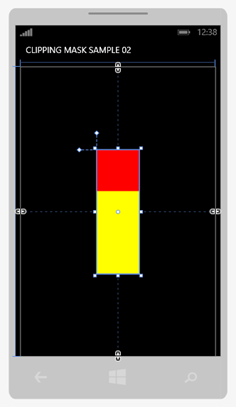We are almost there. Now that we know how to move the clipping mask, we can animate it. There are several ways we can animate objects. We can do it by code, we can use bindings to bind the properties to other controls like a scroller or a slider.

For this example, lets animate it using triggers, so we can keep all the code at the XAML level. There are a few things to note (check the links on each step for detailed documentation on MSDN).

1. First, we want the Y property of the TranslateTransform element to start at 0, so lets change that to 0 again.
2. Next, since we are going to animate the Y property of the transform, we need to give the transform a name we can use to reference it within the trigger’s animation.
3. Then, we can add the trigger to the rectangle. In this case we will use an EventTrigger for the Loaded event.
4. Lets add the storyboard code to the trigger. Use a DoubleAnimation so we can animate the transform’s Y property (which is of type double).
5. You can use the RepeatBehavior and the AutoReverse properties so the animation plays in a loop from beginning to end, and then rewinds itself automatically.

Here’s the final code, including the main Grid container:

`  1: <!--ContentPanel - place additional content here-->  2: <Grid x:Name="ContentPanel" Grid.Row="1" Margin="12,0,12,0">  3:   4:     <Rectangle HorizontalAlignment="Center"  5:                 VerticalAlignment="Center"  6:                 Fill="Red"  7:                 Height="300"  8:                 Width="100"/>  9:  10:     <Rectangle HorizontalAlignment="Center" 11:                 VerticalAlignment="Center" 12:                 Fill="Yellow" 13:                 Height="300" 14:                 Width="100"> 15:         <Rectangle.Clip> 16:             <RectangleGeometry Rect="0, 0, 100, 300"> 17:                 <RectangleGeometry.Transform> 18:                     <TranslateTransform x:Name="MaskTransform"  19:                                         Y="0" /> 20:                 </RectangleGeometry.Transform> 21:             </RectangleGeometry> 22:         </Rectangle.Clip> 23:         <Rectangle.Triggers> 24:             <EventTrigger RoutedEvent="Rectangle.Loaded"> 25:                 <BeginStoryboard> 26:                     <Storyboard> 27:                         <DoubleAnimation  28:                             Storyboard.TargetName="MaskTransform"  29:                             Storyboard.TargetProperty="(TranslateTransform.Y)" 30:                             From="0.0"  31:                             To="300.0"  32:                             Duration="0:0:3"  33:                             RepeatBehavior="Forever"  34:                             AutoReverse="True" /> 35:                     </Storyboard> 36:                 </BeginStoryboard> 37:             </EventTrigger> 38:         </Rectangle.Triggers> 39:     </Rectangle> 40:  41: </Grid>`

So that’s it for this post. I showed you how to animate a clipping mask to achieve nice effects in your Windows Phone applications, all by XAML code!

Here are a few screenshots from the app running on the emulator.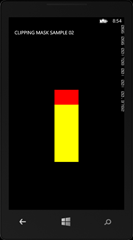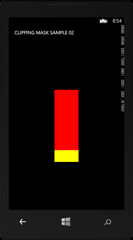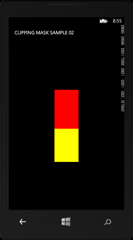In following posts, I’ll share with you different techniques to trigger the animations, using both code behind and XAML animations, and also combining them with bindings, converters and other controls.

I hope it helps you out. Remember, .NET is one of the fastest platforms to prototype and try things. Always start with squares and boxes to put together rapid proofs of concepts. You will be amazed of how quick you can test behaviours, animations and functionality!